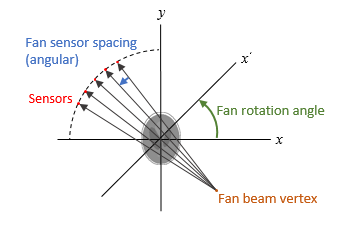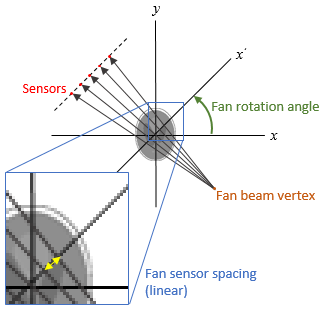Documentation

This is machine translation

Mouseover text to see original. Click the button below to return to the English version of the page.

ifanbeam

Inverse fan-beam transform

Syntax

``I = ifanbeam(F,D)``
``I = ifanbeam(F,D,Name,Value)``
``[I,H] = ifanbeam(___)``

Description

example

````I = ifanbeam(F,D)` reconstructs the image `I` from fan-beam projection data in `F`. Each column of `F` contains fan-beam projection data at one rotation angle. The angle between sensors is assumed to be uniform and equal to the increment between fan-beam rotation angles. `D` is the distance from the fan-beam vertex to the center of rotation.```

example

````I = ifanbeam(F,D,Name,Value)` uses name-value pairs to control various aspects of the reconstruction. Argument names can be abbreviated, and case does not matter.```
````[I,H] = ifanbeam(___)` also returns the frequency response of the filter, `H`.```

Examples

collapse all

Create a sample image. The `phantom` function creates a phantom head image.

`ph = phantom(128);`

Create a fan-beam transformation of the phantom head image.

```d = 100; F = fanbeam(ph,d);```

Reconstitute the phantom head image from the fan-beam representation. Display the original image and the reconstituted image.

```I = ifanbeam(F,d); imshow(ph)``````figure imshow(I);```Create a sample image. The phantom function creates a phantom head image.

`ph = phantom(128);`

Create a radon transformation of the image.

`P = radon(ph);`

Convert the transformation from parallel beam projection to fan-beam projection.

```[F,obeta,otheta] = para2fan(P,100,... 'FanSensorSpacing',0.5,... 'FanCoverage','minimal',... 'FanRotationIncrement',1);```

Reconstitute the image from fan-beam data.

```phReconstructed = ifanbeam(F,100,... 'FanSensorSpacing',0.5,... 'Filter','Shepp-Logan',... 'OutputSize',128,... 'FanCoverage','minimal',... 'FanRotationIncrement',1);```

Display the original and the transformed image.

`imshow(ph)````figure imshow(phReconstructed)```Input Arguments

collapse all

Fan-beam projection data, specified as a numsensors-by-numangles numeric matrix. numsensors is the number of fan-beam sensors and numangles is the number of fan-beam rotation angles. Each column of `F` contains the fan-beam sensor samples at one rotation angle.

Data Types: `double` | `single`

Distance in pixels from the fan-beam vertex to the center of rotation, specified as a positive number. `ifanbeam` assumes that the center of rotation is the center point of the projections, which is defined as `ceil(size(F,1)/2)`. The figure illustrates `D` in relation to the fan-beam vertex for one fan-beam projection.Data Types: `double` | `single`

Name-Value Pair Arguments

Specify optional comma-separated pairs of `Name,Value` arguments. `Name` is the argument name and `Value` is the corresponding value. `Name` must appear inside quotes. You can specify several name and value pair arguments in any order as `Name1,Value1,...,NameN,ValueN`.

Example: ```I = ifanbeam(F,D,'FanRotationIncrement',5)```

Range of fan-beam rotation, specified as the comma-separated pair consisting of `'FanCoverage'` and one of these values.

• `'cycle'` — Rotate through the full range [0, 360) degrees.

• `'minimal'` — Rotate through the minimum range necessary to represent the object.

Fan-beam rotation angle increment in degrees, specified as the comma-separated pair consisting of `'FanRotationIncrement'` and a positive scalar.Data Types: `double`

Fan-beam sensor positioning, specified as the comma-separated pair consisting of `'FanSensorGeometry'` and one of the following values.

Value

Meaning

Diagram

`'arc'`

Sensors are spaced at equal angles along a circular arc at distance `D` from the center of rotation.

`FanSensorSpacing` defines the angular spacing in degrees.`'line'`

Sensors are spaced at equal distances along a line that is parallel to the x' axis. The closest sensor is distance `D` from the center of rotation.

`FanSensorSpacing` defines the distance between fan-beams on the x' axis, in pixels.Fan-bean sensor spacing, specified as the comma-separated pair consisting of `'FanSensorSpacing'` and a positive scalar.

• If `FanSensorGeometry` is `'arc'`, then `FanSensorSpacing` defines the angular spacing in degrees.

• If `FanSensorGeometry` is `'line'`, then `FanSensorSpacing` defines the linear distance between fan-beams, in pixels. Linear spacing is measured on the x' axis.

Data Types: `double`

Filter to use for frequency domain filtering, specified as the comma-separated pair consisting of `'Filter'` and one of the values in the table. For more information, see `iradon`.

Value

Description

`'Ram-Lak'`

Cropped Ram-Lak or ramp filter. The frequency response of this filter is | `f` |. Because this filter is sensitive to noise in the projections, one of the filters listed below might be preferable. These filters multiply the Ram-Lak filter by a window that de-emphasizes high frequencies.

`'Shepp-Logan'`

Multiplies the Ram-Lak filter by a `sinc` function

`'Cosine'`

Multiplies the Ram-Lak filter by a `cosine` function

`'Hamming'`

Multiplies the Ram-Lak filter by a Hamming window

`'Hann'`

Multiplies the Ram-Lak filter by a Hann window

`'None'`No filtering. `ifanbeam` returns unfiltered data.

Data Types: `char` | `string`

Scale factor for rescaling the frequency axis, specified as the comma-separated pair consisting of `'FrequencyScaling'` and a positive number in the range (0, 1]. If `'FrequencyScaling'` is less than 1, then the filter is compressed to fit into the frequency range `[0,FrequencyScaling]`, in normalized frequencies; all frequencies above `FrequencyScaling` are set to `0`. For more information, see `iradon`.

Data Types: `double`

Type of interpolation used between the parallel-beam and fan-beam data, specified as the comma-separated pair consisting of `'Interpolation'` and one of the following values.

`'nearest'` — Nearest-neighbor

`'linear'` — Linear (the default)

`'spline'` — Piecewise cubic spline

`'pchip'` — Piecewise cubic Hermite (PCHIP)

Data Types: `char` | `string`

Size of the reconstructed image, specified as the comma-separated pair consisting of `'OutputSize'` and a positive integer. The image has an equal number of rows and columns.

If you specify `OutputSize`, then `ifanbeam` reconstructs a smaller or larger portion of the image but does not change the scaling of the data.

Note

If the projections were calculated with the `fanbeam` function, then the reconstructed image might not be the same size as the original image.

If you do not specify `OutputSize`, then the size is calculated automatically by:

`OutputSize = 2*floor(size(`R`,1)/(2*sqrt(2)))`

where `R` is the length of parallel-beam projection data used by `iradon`. For more information, see Algorithms.

Data Types: `double`

Output Arguments

collapse all

Reconstructed image, specified as a 2-D numeric matrix.

Frequency response of the filter, returned as a numeric vector.

Data Types: `double`

Tips

• To perform an inverse fan-beam reconstruction, you must give `ifanbeam` the same parameters that were used to calculate the projection data, `F`. If you use `fanbeam` to calculate the projection, then make sure the parameters are consistent when calling `ifanbeam`.

Algorithms

`ifanbeam` converts the fan-beam data to parallel beam projections and then uses the filtered back projection algorithm to perform the inverse Radon transform. The filter is designed directly in the frequency domain and then multiplied by the FFT of the projections. The projections are zero-padded to a power of 2 before filtering to prevent spatial domain aliasing and to speed up the FFT.

 Kak, A. C., and M. Slaney, Principles of Computerized Tomographic Imaging, New York, NY, IEEE Press, 1988.